Chapter 2 National Income - Part 5 Expenditure Method

Economics Class 12
Macroeconomics

#### Example 29

Calculate 'Gross Domestic Product of Factor Cost' from the following data: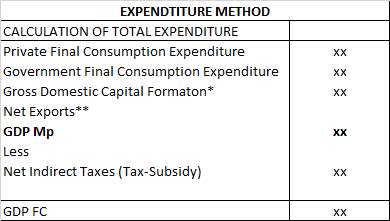In this question

We are given Net Domestics Capital Formation (and not gross)

So we use following Steps

Step 1 Calculate Gross Domestic Capital Formation

Gross Domestic Capital Formation

=Net Domestic Capital formation +Depreciation

=Net Domestic Capital formation +Consumption of Fixed Capital

150 + 50 = 200

Step 2 Calculate GDP FC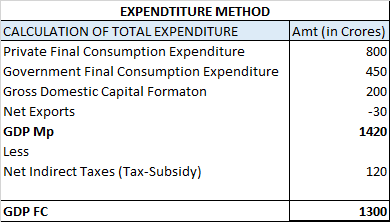#### Example 28

Calculate GNP at FC:

In this question, we have to calculate GNP FC

and not NNP FC

So we will not use depreciation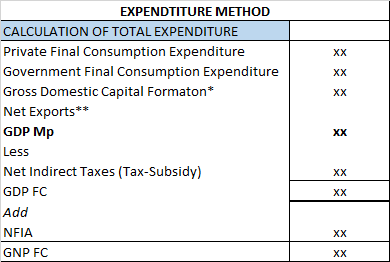In this question

We are given Net Domrstic Fixed Capital Formaton

First we calculate Gross Domestic Fixed Capital formation

and from that,we calculate

Gross Domestic Capital formation

So we use following Steps

Step 1 Calculate Gross Domestic Fixed Capital Formation

=Gross Fixed Capital formation

=Net Fixed Capital formation +Depreciation

=Net Fixed Capital formation +Consumption of Fixed Capital

=350+50

=400

Step 2 Calculate Gross Domestic Capital Formation

Gross Domestic Capital Formation

=Expenditure on Fixed Assets +Expenditure on Stock

=Gross Fixed Capital formation + Inventory Investment

=Gross Fixed Capital formation +(Closing Stock-Opening Stock)

400 + (100 - 60 ) = 440

Step 3 Calculate GNP FC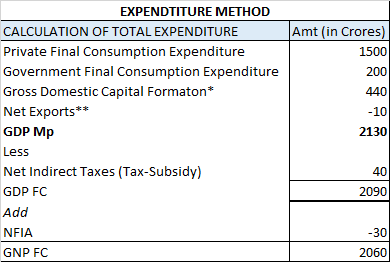#### Question 28

Calculate NDP at FC.

In this question, we need to calculate NDP at FC

So we use following format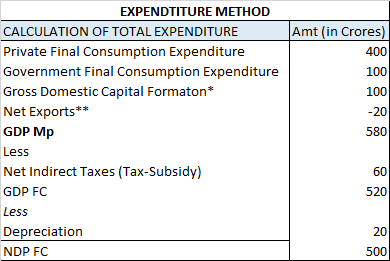#### Question 27

Calculate GNP at MP.

In this question, we need to calculate GNP MP

So we use following format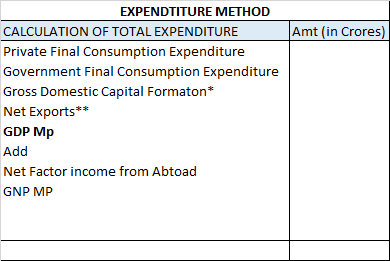#### Note

Personal final consumption Expenditure and Persona Consumption expenditure are Sam things

Govt Final Consumption Expenditure and Govt Consumption expenditure are different things

But we need to calculate Gross domestic Capital formation and Nest Exports

Step 1 We calculate Net Exports

Net Exports

=Exports of goods and servives -Imports of goods and services

450 - 500 = -50

Step 2 We calculate Gross Domestic Capital Formation

* Gross Domestic Capital Formation

=Expenditure on Fixed Assets +Expenditure on Stock

=Gross Fixed Capital formation + Inventory Investment

=2500+ Inventory Investment

=2500+ (Closing Stock-Opening Stock)

=2500+ (Increase in Stock)

=2500 -Fall in Stock

=2500 -300

=2200

Step 3

We calculate GNP MP using this format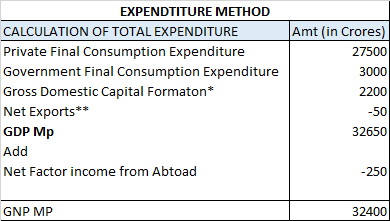Learn in your speed, with individual attention - Teachoo Maths 1-on-1 Class